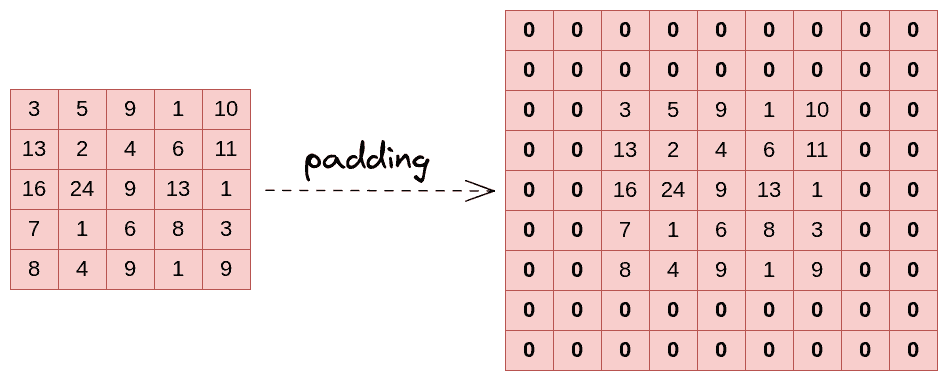## 1. Introduction

In this tutorial, we’ll walk through padding, a machine-learning technique widely used that processes the input data and improves the performance and accuracy of the model. In particular, we’ll introduce padding, explain its usage, highlight its importance, and give a brief example of how it works.

In machine learning and in neural networks, convolutional filters are applied to images in order to extract useful information from them, such as patterns, edges, and corners. Since animage convolves with afilter, the output’s dimension is. Due to the convolutional operation, the image shrinks, especially if the neural network is quite deep, with many layers. Furthermore, in contrast to the center pixels, which are engaged in numerous convolutional areas, corner pixels are employed less often and are not as much involved when applying a convolutional operation to a matrix. This causes the edges of the input picture to lose relevant information. Padding comes to deal with these issues.

Padding is a technique employed that guarantees that the input data has the exact size and shape that the model anticipates after every convolutional operation at each stage of the deep learning model. So this is ensured by adding additional elements to the input data, such as zeros, to make it conform to the model’s specifications:## 3. Different Types of Padding

Same Padding: This type of padding adds an equal number of elements (), typically zeros, to the top, bottom, left, and right sides of the inputimage so that the output size is the same as the input size.

Valid Padding: This kind of padding computes the input matrix’s valid elements rather than adding new ones. Therefore, the output size is less than the input size. Valid padding denotes that there is no padding and that all of the proportions are accurate, resulting in a filter that completely covers the input picture.

Causal Padding: This type of padding is used in sequence-to-sequence models and time series forecasting and basically works with the one-dimensional convolutional layers. Causal padding adds elements to the start of the data, which also aids in forecasting the values of early time steps.

Note that the choice of padding can significantly impact the model’s performance and accuracy.

## 4. Why Is Padding Used?

Padding is one of the most crucial machine learning techniques since it allows for the modification of input size in order to be compatible with the neural network’s requirements. It adds elements to the input matrix before any convolutional filter is applied, and thus, it aids in preventing any information loss, particularly from the edges of the image. Moreover, padding helps to prevent the image’s compression after every convolutional operation.

Lastly, it aids in maintaining the spatial relationship and characteristics of the input data and can significantly impact the model’s overall performance.